# How to perform Conditional Formatting with formula in Excel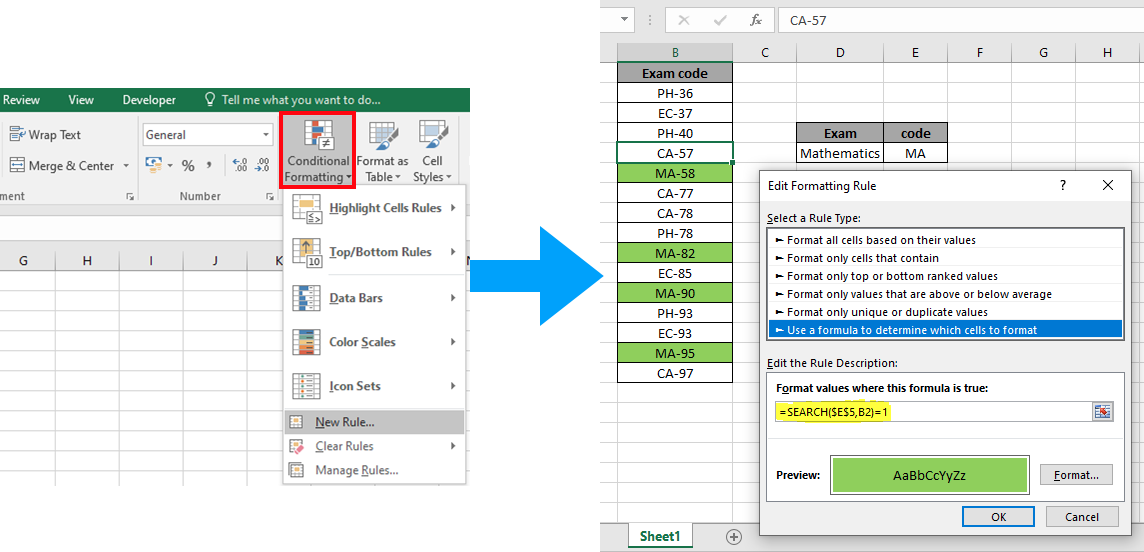In this article, we will learn How to perform Conditional Formatting with formula in Excel.

Why do we use conditional formatting to highlight cells in Excel ?

Conditional Formatting is used to highlight the data on the basis of some criteria. It would be difficult to see various trends just for examining your Excel worksheet. Conditional Formatting provides a way to visualize data and make worksheets easier to understand. It allows you to apply the formatting basis on the cell values such as colours, icons and data bars.

Excel Conditional formatting gives you inbuilt formulation like Highlight cells rules or top/bottom rules. These will help you achieve formulation using simple steps.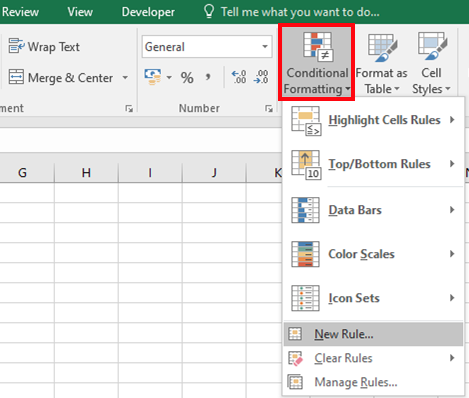Choose the New rule to create a customized formula for highlighting cells. For example Highlight sales values where sales values are in between 150 to 1000. For this we use Conditional formatting. Try the formula in adjacent cells before applying in the conditional formatting formula box.

Formula box in Conditional formatting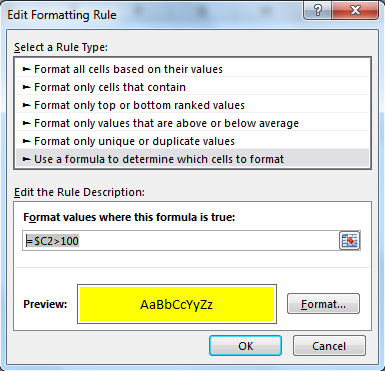After selecting the New rule in conditional formatting. Select the Use a formula to determine which cells to format -> it will open the formula box as shown in the image above. Use the formula in it and select the type of formatting to choose using Format option (like Yellow background as shown in the image).

How to solve the problem?

For this we will be using the Conditional formatting obviously. And we can use any of the two functions SEARCH function or FIND function. The difference is SEARCH function, is case insensitive which means it will match any of  substrings "ABC", "Abc", "aBc", "ABc", if matched with "abc". FIND function is case sensitive which matches only substring "abc", if matched with "abc". Below shown is the generic formula to solve this problem.

SEARCH Formula Syntax:

 =SEARCH(sp_val, cell)=1

FIND Formula Syntax:

 =FIND(sp_val, cell)=1

sp_val : specific or particular value to match with.

cell : cell to check

Example :

All of these might be confusing to understand. Let's understand it better via running the formula on some values. Here we have some university exam codes. We need to highlight the mathematics exam codes. Mathematics Exam code begins with "MA".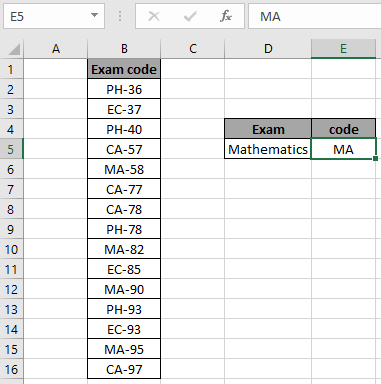What we need here is to check each cell which starts with "MA".

Go to Home -> Conditional formatting -> New Rule

A dialog box appears in front select Use a formula to determine which cells to format -> input the below formula under the Format values where this formula is True:

Use the formula:

 =SEARCH(\$E\$5,B2)=1

Explanation:

1. Search matches the string in the cell value.
2. Search function returns the position of occurrence of the substring in text.
3. If the position matches with 1 i.e. the start of the cell. The formula returns True if matched or else False.
4. True value in conditional formatting formats the cell as required.
5. Customize the cell format by clicking the Format button in the right bottom of the dialog box as shown below.
6. The format will will displayed adjacent to the Format button.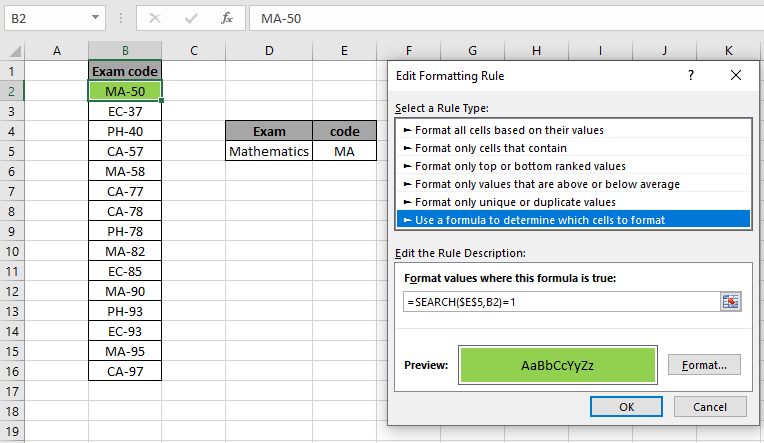The background color gets Green ( highlight formatting) as you click Ok as shown above. Now copy the formula to the rest of cells using the Ctrl + D or dragging down form right bottom of B2 cell.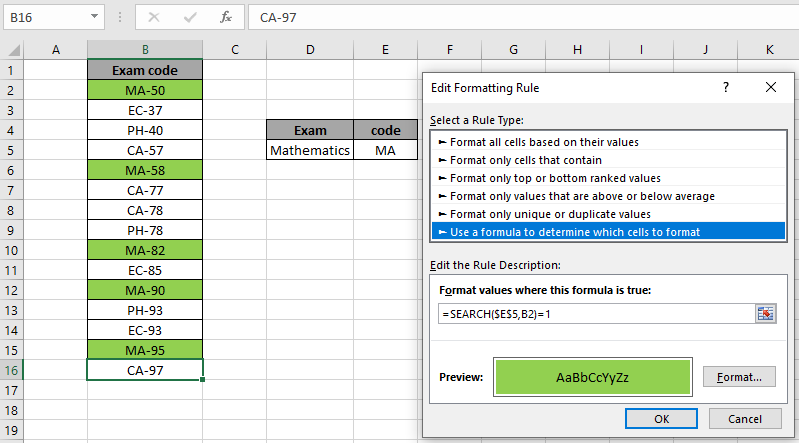As you can see all the mathematics exam codes are highlighted. You can input specific value substring, with quotes(") in the formula along with an example solved using the FIND function as shown below.

FIND function is case sensitive function :

FIND function returns the position of the occurrence of the find_text value in the cell value. The difference occurs if the value you are trying to match must be case sensitive. See the below formula and snapshot to understand How to highlight cells that begin with specific value in Excel.

Use the formula :

 =FIND("MA",B2)=1

B2 : within_text

"MA" : find_text substring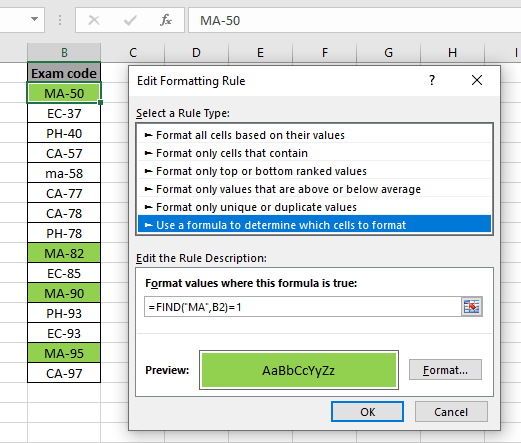As you can see the lowercase exam code "ma-58" doesn't match the substring "MA" as we used the FIND function here. So, it's preferable to use the SEARCH function with conditional formatting. Conditional Formatting provides a way to visualize data and make worksheets easier to understand. You can perform various tasks using the different features of conditional formatting in Excel.

Here are all the observational notes regarding using the formula.

Notes:

1. Conditional formatting allows you to apply the formatting basis on the cell values such as colours, icons and data bars.
2. The formula works for text and numbers both.
3. SEARCH function is case insensitive function.

Hope you understood How to perform Conditional Formatting with formula in Excel. Explore more articles on Excel matching and highlighting cells with formulas here. If you liked our blogs, share it with your friends on Facebook. And also you can follow us on Twitter and Facebook. We would love to hear from you, do let us know how we can improve, complement or innovate our work and make it better for you. Write to us at info@exceltip.com.

Related Articles:

Find the partial match number from data in Excel : find the substring matching cell values using the formula in Excel

How to Highlight cells that contain specific text in Excel : Highlight cells based on the formula to find the specific text value within the cell in Excel.

Conditional formatting based on another cell value in Excel : format cells in Excel based on the condition of another cell using some criteria.

IF function and Conditional formatting in Excel : How to use IF condition in conditional formatting with formula in excel.

Perform Conditional Formatting with formula 2016 : Learn all default features of Conditional formatting in Excel

Conditional Formatting using VBA in Microsoft Excel : Highlight cells in the VBA based on the code in Excel.

Popular Articles :

How to use the IF Function in Excel : The IF statement in Excel checks the condition and returns a specific value if the condition is TRUE or returns another specific value if FALSE.

How to use the VLOOKUP Function in Excel : This is one of the most used and popular functions of excel that is used to lookup value from different ranges and sheets.

How to use the SUMIF Function in Excel : This is another dashboard essential function. This helps you sum up values on specific conditions.

How to use the COUNTIF Function in Excel : Count values with conditions using this amazing function. You don't need to filter your data to count specific values. Countif function is essential to prepare your dashboard.

Terms and Conditions of use

The applications/code on this site are distributed as is and without warranties or liability. In no event shall the owner of the copyrights, or the authors of the applications/code be liable for any loss of profit, any problems or any damage resulting from the use or evaluation of the applications/code.